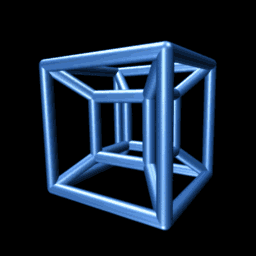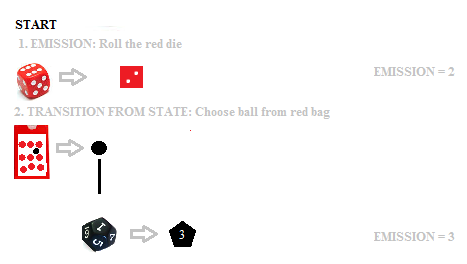# Markov Chain Monte Carlo (MCMC): Non-Technical Overview in Plain English

Markov Chain Monte Carlo is a way to sample from a complicated distribution. What is a “complicated distribution”? One that’s difficult, or near impossible to find probabilities for. These types of problems are called “high-dimensional” problems. One example of a high-dimensional problem is calculating the relative importance of pages on the World Wide Web. Not only are their billions of pages, but they are in a constant state of change. Markov Chain Monte Carlo can solve these types of problems in a reasonable amount of time.

Markov Chain Monte Carlo isn’t a specific technique: it’s actually a large class of sampling algorithms that includes:
The Metropolis algorithm,
Gibbs sampling,
Slice Sampling.

## What is “High Dimensional”?

The key to understanding MCMC is to understand high dimensional space. It’s an entire chapter in some computer science books, so I won’t go into more than just a brief overview here.A hypercube.Basically, high-dimensional space is anything beyond the 2-D and 3-D world we’re used to dealing with. High-dimensional space doesn’t behave the way we think it should. For example, a 4-dimensional cube, the hypercube, collapses in on itself as it rotates. Similarly, a high-dimensional unit-radius sphere has a volume of zero; Cubature (numerical integration) is needed to find these volumes. A high dimensional math problem has data that lies on high-dimensional spaces. Therefore, the data doesn’t “behave” the way we think it should. High-dimensions are difficult to comprehend (it’s said that even Einstein had trouble visualizing hypercubes, although he did perform the math behind four and five dimensions) and their space increases exponentially with each additional dimension–making it practically impossible to add any numerical meaning to the results. It follows then, that finding probabilities for high-dimensional data is extremely difficult. The usual probability rules don’t apply, so new ones are needed, like MCMC.

Note: If you’re interested in finding out more about high-dimensional space, I recommend reading the first couple of chapters in Foundations of Data Science (downloadable pdf — free).

## Markov ChainsAn example of a Markov Model, where dice represent states and colored balls are used to transition between states.

Markov chains are used to model probabilities, where all of the information needed to predict future events can be encoded in the current state. You can find a simple, step-by-step example, using two dice to make predictions, in the Hidden Markov Model article. Basically, two colored die represent the current state (red or black) and a colored ball chosen from a bag gives the probability of transitioning to the next state. In real life, the number of states is likely to be much higher than just two! Hopcraft gives the concrete example of speech, where the last k syllables uttered by the speaker can be analyzed, giving the probability of what the next word might be. The speech is moving from one state to another in such a way that the next word depends only upon the current state (i.e. what’s being said at the moment). The number of possible states for the last k-syllables could reach into the millions, which would be impossible to analyze by hand (or even with a standard computer algorithm).

More technically, this information can be placed into a matrix and a vector(a column matrix) called probability vectors. Many, many, many iterations later and you have a collection of probability vectors…making up the Markov chains.

The purpose of the Markov Chain Monte Carlo is to sample a very large sample space, one that contains googols of data items. One example of such a sample space is the World Wide Web. Analyzing the web for important of pages is behind search engines like Google, and they use Markov chains as part of their analytics. Essentially, they send a bot out on (a literal) random walk of the web, following links wherever they go. These random walks are logged in a matrix, along with probabilities of reaching a certain page.

## Why Markov Chain Monte Carlo?

OK, so we have a complicated distribution — like the world wide web. MCMC is a way to efficiently sample from this complicated distribution. Simple random sampling or similar methods (like picking a name from a hat) won’t work, because you won’t be able to easily define your population because it’s growing exponentially. Even if you did find a way to define the pages on the current WWW, the millions of pieces of new content generated in one minute from now will have a zero probability of being chosen. From a statistical standpoint, this is unacceptable. Enter Markov Chain Monte Carlo.

The “Monte Carlo” part of MCMC is just a moniker for the type of sampling we’re doing — which is sampling based on random walks. Monte Carlo is a gambling resort in Monaco, so it could just as easily have been called “Markov Chain Las Vegas” or just “Markov Chain Sampling.”

## MCMC and Limiting Distributions

MCMC uses random walks (essentially a huge random number generator) to estimate probabilities with the same limiting distribution as the random variable you’re trying to simulate. As time n > ∞, a Markov chain has a limiting distribution π = (πj)j∈s. A value for π can be found by solving a series of linear equations.

References:
Blum, J. et. al. (2011). Foundations of Data Science. Retrieved August 11, 2017 from:https://www.cs.cornell.edu/jeh/bookMay2015.pdf
Agresti A. (1990) Categorical Data Analysis. John Wiley and Sons, New York.
Jerrum, M., & Sinclair, A. (1996). The Markov chain Monte Carlo method: an approach to approximate counting and integration. In D. S. Hochbaum (Ed.), Approximation algorithms for NP-hard problems (pp. 482–519). PWS Publishing.
Kotz, S.; et al., eds. (2006), Encyclopedia of Statistical Sciences, Wiley.

CITE THIS AS:
Stephanie Glen. "Markov Chain Monte Carlo (MCMC): Non-Technical Overview in Plain English" From StatisticsHowTo.com: Elementary Statistics for the rest of us! https://www.statisticshowto.com/markov-chain-monte-carlo/